# Finding Multiple Missing Elements in an Array in C

## Finding Multiple Missing Elements in a Sorted Array in C:

In this article, I am going to discuss Finding Multiple Missing Elements in a Sorted Array in C Language with Examples. In our previous article, we have seen how to find a single missing element in a sorted array.

##### How to Find Multiple Missing Elements in a Sorted Array?

We have an array of some missing elements that we have to find: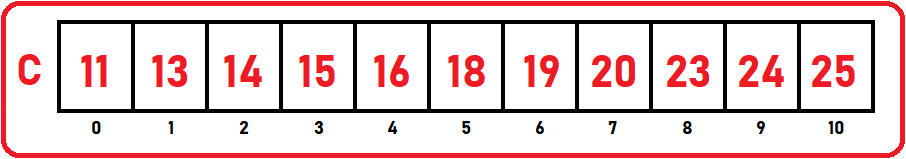In the above array, there are 3 elements missing which are 12, 17, 22, and 22. So here more than one element is missing and also after 20 continuously 2 elements are missing. Here also we will follow the same method which we discuss in our previous article. We will iterate all the elements of an array by checking the difference between element and index.

##### 1st Missing Element:

The difference of 1st element to the corresponding index (0) is: 11 – 0 = 11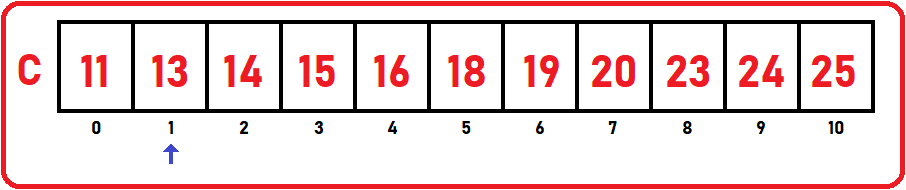2nd element to the corresponding index (1) is 13 – 1 = 12

12 is not the same as the previous difference, which means there is a missing element. We will find that missing element by adding the difference to that corresponding index number, so, 11 is the difference and index number is 1, so by adding them we will get 12. It is our first missing element. We know that there are more missing elements, so we can’t track the whole array with the same difference. We have to modify the difference when we found any missing element. Here the new difference will 12.

##### 2nd Missing Element:

Now the new difference is 12. Let iterate from where we left in the array.

The difference of:

3rd element to the corresponding index (2) is: 14 – 2 = 12

4th element to the corresponding index (3) is 15 – 3 = 12

5th element to the corresponding index (4) is: 16 – 4 = 12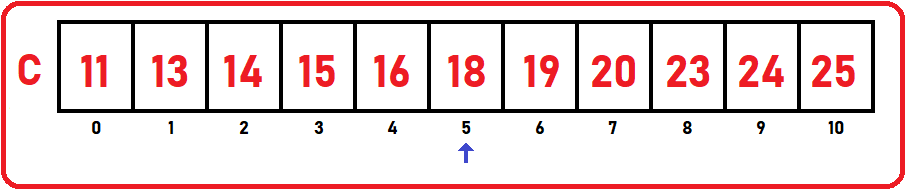6th element to the corresponding index (5) is 18 – 5 = 13

Here, 12 is not the same as the previous difference, there is a missing element. So again, for finding the missing element we will repeat the step. Now, add the difference and index number. 12 is the difference and 5 is the index number, so by adding them we will get 17. So, 17 is the 2nd missing element. Now the new difference is 13.

##### 3rd and 4thMissing Elements:

Now the new difference is 13. Let iterate from where we left in the array.

The difference of:

7th element to the corresponding index (6) is 19 – 6 = 13

8th element to the corresponding index (7) is: 20 – 7 = 13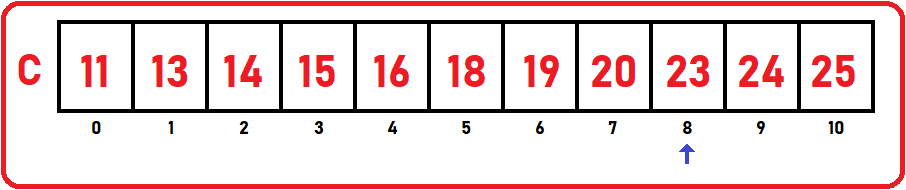9th element to the corresponding index (8) is 23 – 8 = 15

Here, 15 is not the same as the previous difference, here 15 – 13 is 2 means there are two missing elements. So again, for finding the missing element we will repeat the step. Now, our difference is 13, so,

13 + 8 = 21,

Increment difference by 1 then,

14 + 8 = 22

So, 21 and 22 are our 3rd and 4th continuously missing elements. When the difference will greater than one, we will increment the index (current index to the new index). We can continue further but as we know there are no more missing elements in the array so we have left here. In this way, we can find multiple missing elements in a sequence of elements or an array.

##### Pseudo Code:
```for (i = 0; i < n; i++){
if(C[i] - i != difference){
while(difference < C[i] - i){
printf("%d\n", i + difference);
difference++;
}
}
}
```

While loop is used in the above code because we have to print missing elements and increment the difference if there is a difference greater than 1.

##### Full code in C language:
```#include <stdio.h>
#include <stdlib.h>

struct List{
int C;
int size;
int length;
};

void Display(struct List list) {
int i;
printf("Elements are:\n");
for (i = 0;i<list.length;i++)
printf("%d ", list.C[i]);
printf("\n\n");
}

void MissingElements(struct List list){
int diff = list.C;

printf("Missing Elements are: \n");
for (int i = 0; i < list.length; i++){
if(list.C[i] - i != diff){
while(diff < list.C[i] - i){
printf("%d\n", i + diff);
diff++;
}
}
}
}

int main(){
struct List list_1 = {{11,13,14,15,16,18,19,20,23,24,25}, 15, 11};

Display(list_1);

MissingElements(list_1);
}
```

Time Complexity: O (n)

###### Output: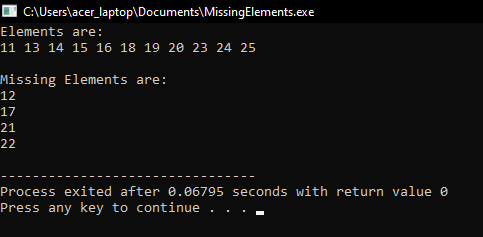##### Finding Multiple Missing Elements in Array Method (2nd Method):

Now, we will see another method to find out multiple missing elements or sequences of elements in an array (2nd Method). This method is the faster method as compared to the previous method. So below is an example of array ‘C’ which has the lowest element: 1, highest element: 12, and size are 10: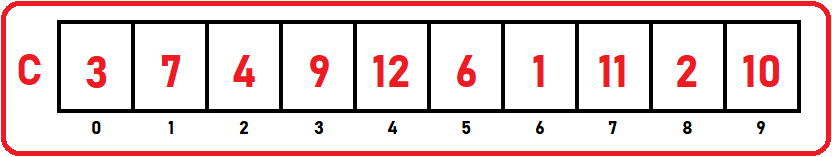By noticing the above array, there are all numbers present from 1 to 12 but 5 and 8 are missing. Our previous method takes O (n) time with some extra time for increment difference variable but there we neglect this time as it was too small. But this method takes O (n) time without any extra time.

For making this method faster, we have taken an extra array which index is from 0 to 12. In this method, we will take the size of the second array as the largest element present in the given array. Here, 12 is the max number so we have taken an extra array of size 12: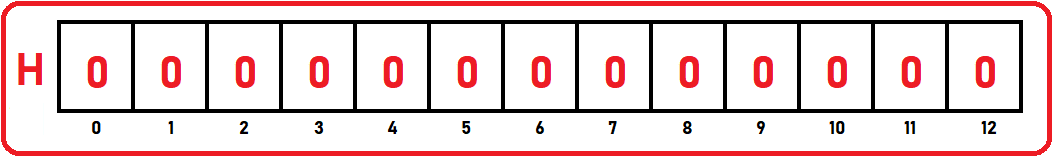We have initialized the extra array with 0. Why do we initialize with 0? Let’s have a look at the procedure:

We have to scan through the array one by one element.

##### 1st Element:In the given array, 1st element is 3. Now, go to the 3rd index of the extra array and increment the value of the 3rd index: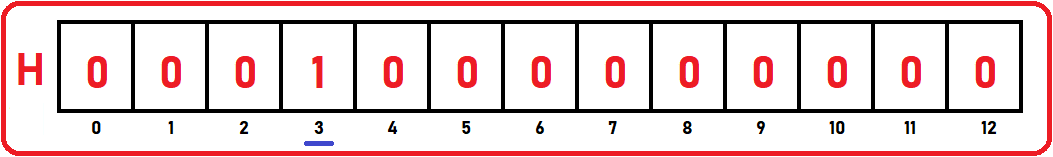##### 2nd Element: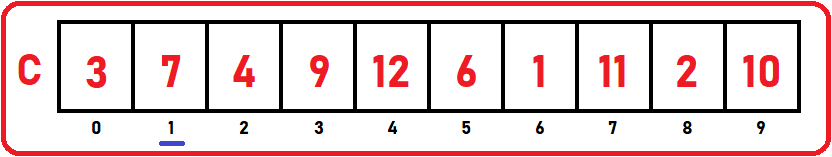Now the next is the 2nd element which is 7. Now, go to the 7th index of the extra array and increment the value of the 7th index: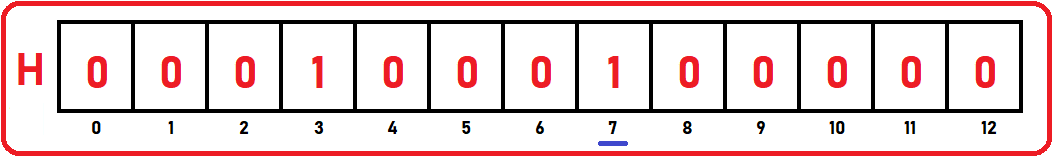##### 3rd Element: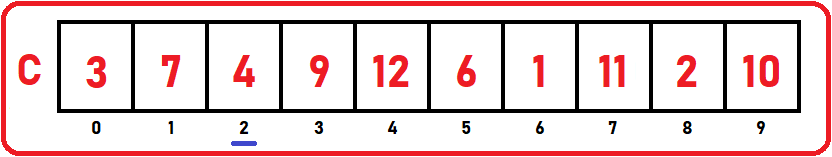Now the next is 3rd element which is 4. Now, go to the 4th index of the extra array and increment the value of the 4th index: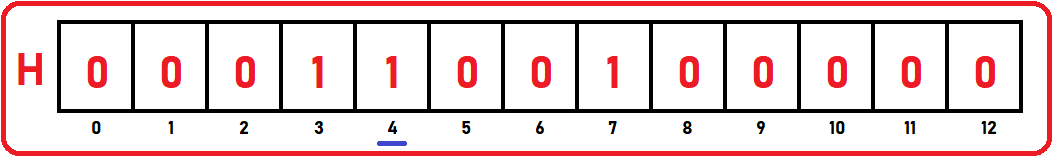In this way, we have traced the whole array and modified the extra array as: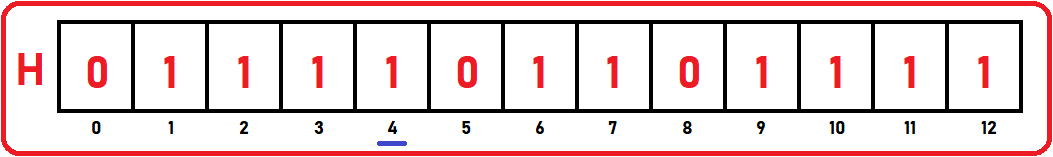So, what do we do? We just increment that particular index that is present in the given array. So zero are that indices that are not present in the given array and one is those indices that are present in our array. Now our extra array has 3 indexes of zero elements which are: 0, 5, and 8. Here we will neglect zero because it is not present in the given array as our array start from 1. Still, there are two places that are zero. So, these two elements are missing.

Now we will scan the extra array and print only those elements which have the value of zero. This is a simple and faster procedure. As we just scanned the list of elements and increment the indices of the extra array. So, for incrementing the value it just takes constant time and for scanning, it will take n time, so,

Time Complexity: O (n)

Here we named our extra array ‘H’ and it is known as Hash Table. We will discuss the hash table later in our upcoming articles. But here we did a simple implementation of hast table. As we scan the array one by one element, we just go to the same index and increment it.

Always remember hast table takes constant time. That is the ideal time for hashing is constant. We can also call it a bitset. Here we haven’t implemented the complete mechanism of hashing technique like using a hash function and other things. But it is the simplest form of hashing. Here, we need an array space equal to the largest element. So extra array can take a lot of space. So, space, if it is a constraint then you cannot use this hashing (if you have limited space).

But the machines that we are using have abandoned the memory space, so we hardly bother about memory space. So hashing is the best solution.

##### Full Code in C language:
```#include <stdio.h>
#include <stdlib.h>

struct List{
int C;
int size;
int length;
};

void Display(struct List list) {
int i;
printf("Elements are:\n");
for (i = 0;i<list.length;i++)
printf("%d ", list.C[i]);
printf("\n\n");
}

void MissingElements_2(struct List list, int low, int high, int size){

int H[size];
for(int i = 0; i < size; i++){
H[i] = 0;
}

for(int i = 0; i < list.length; i++){
H[list.C[i]]++;
}

printf("Missing Elements are: \n");
for (int i = low; i <= high; i++){
if(H[i] == 0)
printf("%d\n", i);
}
}

int main(){
struct List list_1 = {{3, 7, 4, 9, 12, 6, 1, 11, 2, 10}, 10, 10};

Display(list_1);

MissingElements_2(list_1, 1, 12, 10);
}
```
###### Output: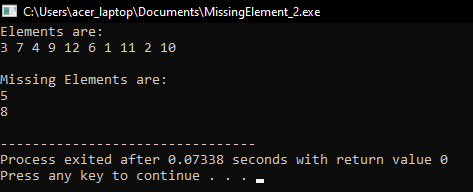In the next article, I am going to discuss Finding Duplicates in a Sorted Array in C Language with Examples. Here, in this article, I try to explain How to Find Multiple Missing Elements in a Sorted Array in C Language with Examples and I hope you enjoy this Finding Multiple Missing Elements in a Sorted Array in C Language with Examples article.